Physical Chemistry Reference Physical Chemistry Principles and Applications

• Slides: 48Physical Chemistry Reference: Physical Chemistry Principles and Applications in Biological Sciences Tinoco and others Fourth Edition-2002 By Dr. Gehan Abdel Raouf 1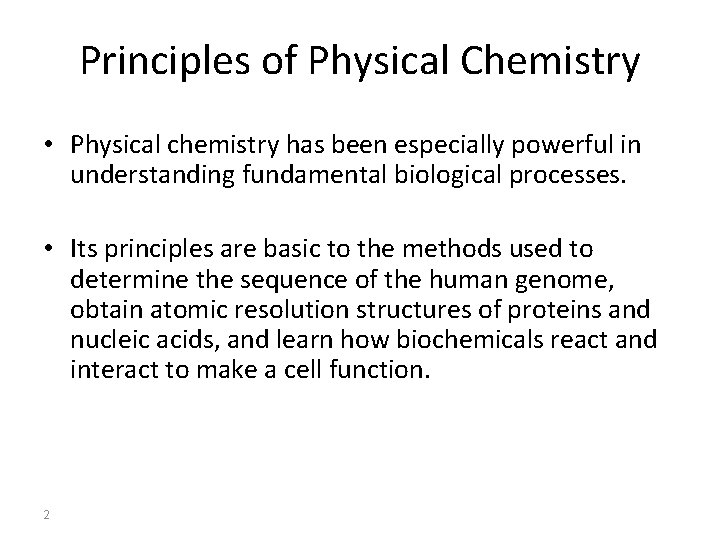Principles of Physical Chemistry • Physical chemistry has been especially powerful in understanding fundamental biological processes. • Its principles are basic to the methods used to determine the sequence of the human genome, obtain atomic resolution structures of proteins and nucleic acids, and learn how biochemicals react and interact to make a cell function. 2Course Portfolio • Thermodynamics • Intermolecular forces • Quantum mechanics and its biological application • Chemistry of radiation 3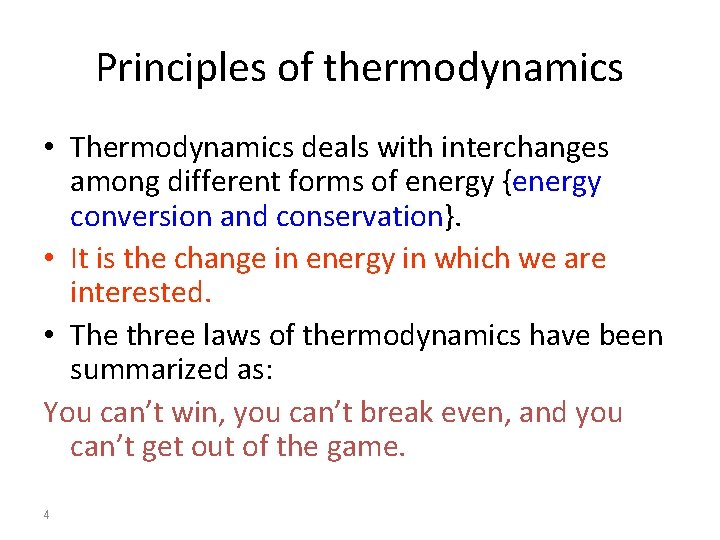Principles of thermodynamics • Thermodynamics deals with interchanges among different forms of energy {energy conversion and conservation}. • It is the change in energy in which we are interested. • The three laws of thermodynamics have been summarized as: You can’t win, you can’t break even, and you can’t get out of the game. 4Thermodynamics • Thermodynamics originated as the study of the relationship between mechanical and thermal energy- the energy associated with the disordered motions of the atoms and molecules within a substance • It is important whenever temperature play a role. 5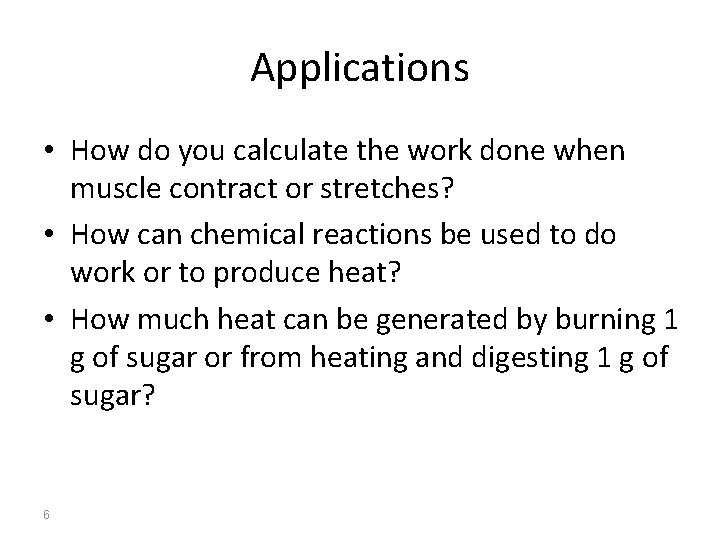Applications • How do you calculate the work done when muscle contract or stretches? • How can chemical reactions be used to do work or to produce heat? • How much heat can be generated by burning 1 g of sugar or from heating and digesting 1 g of sugar? 6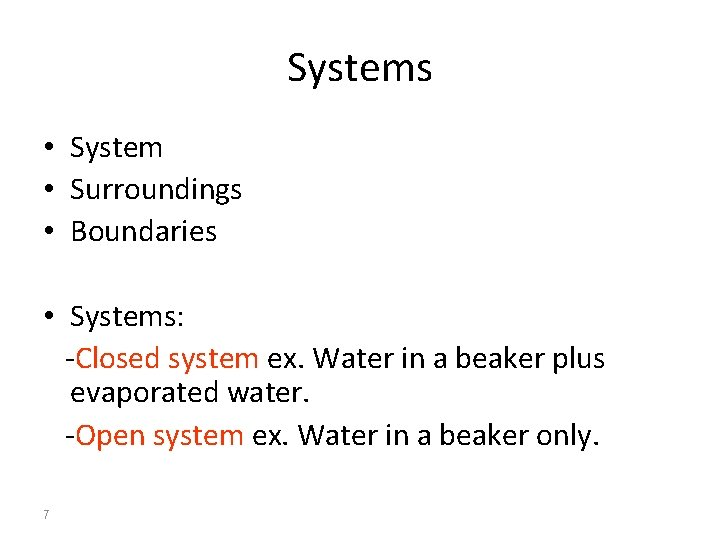Systems • System • Surroundings • Boundaries • Systems: -Closed system ex. Water in a beaker plus evaporated water. -Open system ex. Water in a beaker only. 7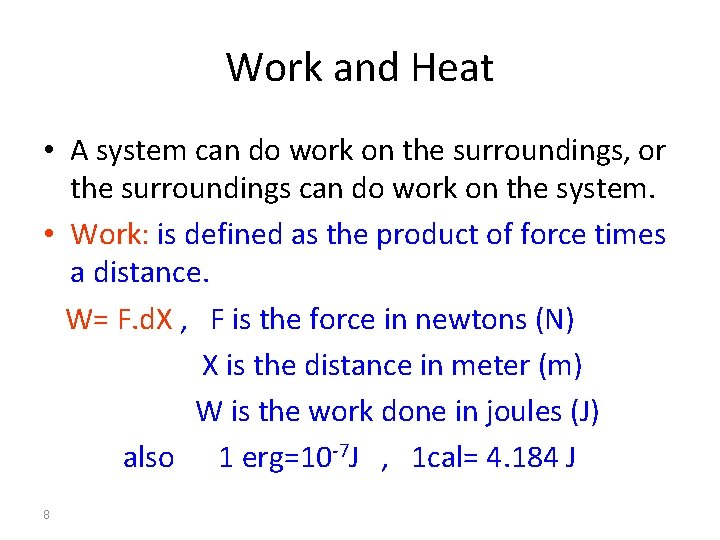Work and Heat • A system can do work on the surroundings, or the surroundings can do work on the system. • Work: is defined as the product of force times a distance. W= F. d. X , F is the force in newtons (N) X is the distance in meter (m) W is the work done in joules (J) also 1 erg=10 -7 J , 1 cal= 4. 184 J 8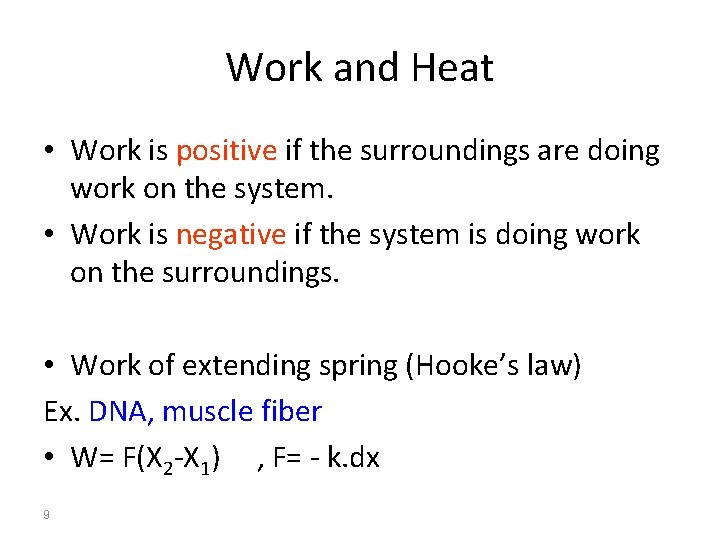Work and Heat • Work is positive if the surroundings are doing work on the system. • Work is negative if the system is doing work on the surroundings. • Work of extending spring (Hooke’s law) Ex. DNA, muscle fiber • W= F(X 2 -X 1) , F= - k. dx 9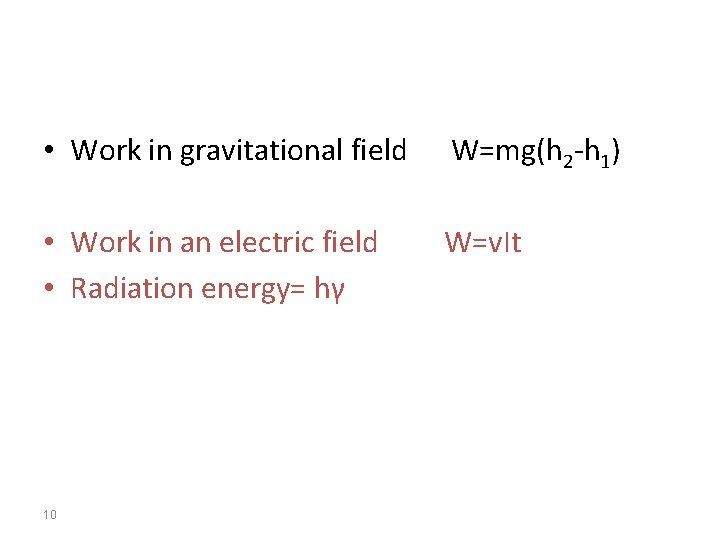• Work in gravitational field W=mg(h 2 -h 1) • Work in an electric field • Radiation energy= hγ W=v. It 10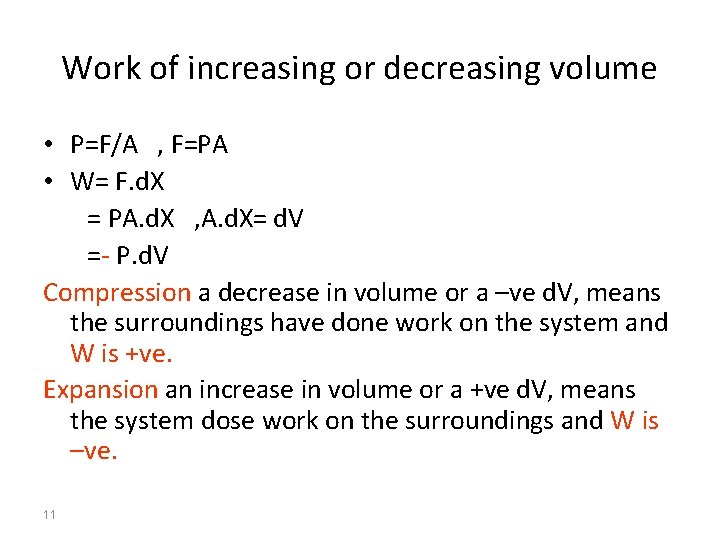Work of increasing or decreasing volume • P=F/A , F=PA • W= F. d. X = PA. d. X , A. d. X= d. V =- P. d. V Compression a decrease in volume or a –ve d. V, means the surroundings have done work on the system and W is +ve. Expansion an increase in volume or a +ve d. V, means the system dose work on the surroundings and W is –ve. 11Ex. 2. 2 P 22 • Calculate the PV work done when a system containing a gas expands from 1. 0 L to 2. 0 L against a constant external pressure of 10 atm. {1 L. 1 atm. = 101. 325 J} • W = -P(V 2 -V 1) =-10(2 -1) = -10 L. atm W =-10 x 101. 325 = -1013 J the system dose work; W is negative. 12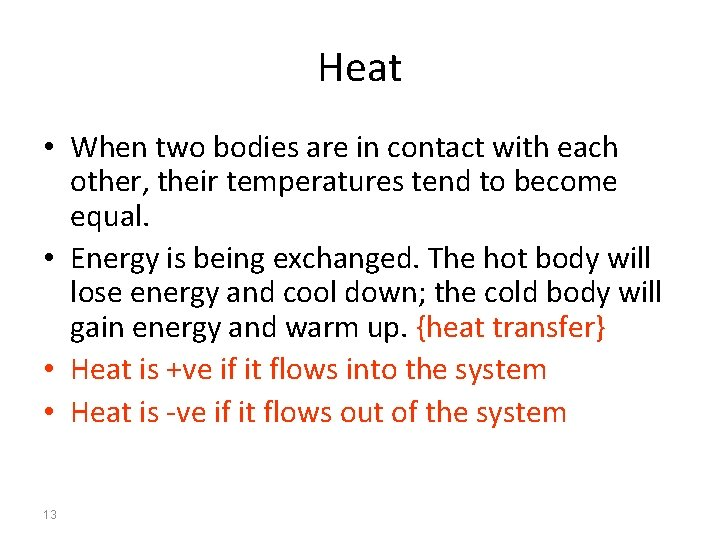Heat • When two bodies are in contact with each other, their temperatures tend to become equal. • Energy is being exchanged. The hot body will lose energy and cool down; the cold body will gain energy and warm up. {heat transfer} • Heat is +ve if it flows into the system • Heat is -ve if it flows out of the system 13• For closed system, the transfer of a small quantity of heat dq will result in a change d. T in its temperature. • The ratio dq/d. T is called heat capacity C C = dq/d. T → q= C(T 2 -T 1) Heat capacity of every material: is a quantity that characterizes how much heat is necessary to raise its temperature by 1 o. C or 1 K. It is usually determined by measuring the changes in temperature when known amounts of electrical energy are dissipated in this material. Its units are JK-1 mol-1 K = o. C + 273. 15 14 or cal K-1 mol-1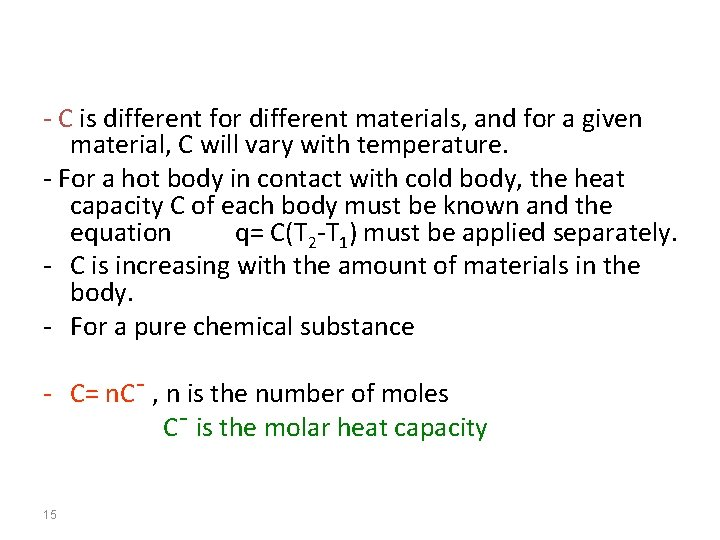- C is different for different materials, and for a given material, C will vary with temperature. - For a hot body in contact with cold body, the heat capacity C of each body must be known and the equation q= C(T 2 -T 1) must be applied separately. - C is increasing with the amount of materials in the body. - For a pure chemical substance - C= n. C¯ , n is the number of moles C¯ is the molar heat capacity 15• Ex. 2. 5 p 26 • Calculate the heat, in joules, necessary to change the temperature of 100 g of liquid water by 50 o. C at constant pressure. The heat capacity of liquid water at constant pressure is 1 cal g-1 deg-1 and is nearly independent of temperature. (1 cal= 4. 184 J) • The heat absorbed by the system • q=C(T 2 -T 1) • = 100 g (1 cal g-1 deg-1 )x(50 deg)x 4. 184 • =20. 9 KJ 16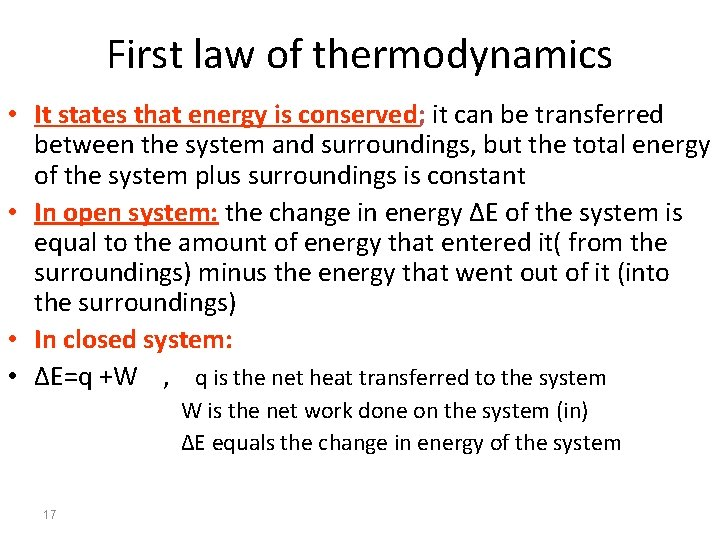First law of thermodynamics • It states that energy is conserved; it can be transferred between the system and surroundings, but the total energy of the system plus surroundings is constant • In open system: the change in energy ΔE of the system is equal to the amount of energy that entered it( from the surroundings) minus the energy that went out of it (into the surroundings) • In closed system: • ΔE=q +W , q is the net heat transferred to the system W is the net work done on the system (in) ΔE equals the change in energy of the system 17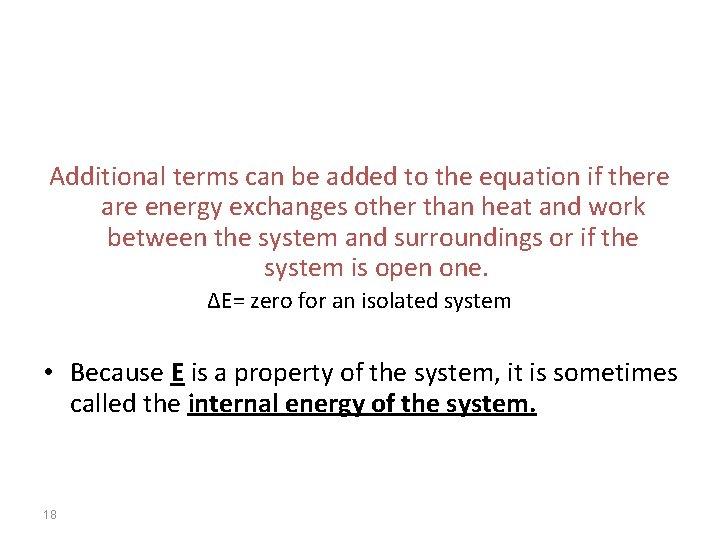Additional terms can be added to the equation if there are energy exchanges other than heat and work between the system and surroundings or if the system is open one. ΔE= zero for an isolated system • Because E is a property of the system, it is sometimes called the internal energy of the system. 18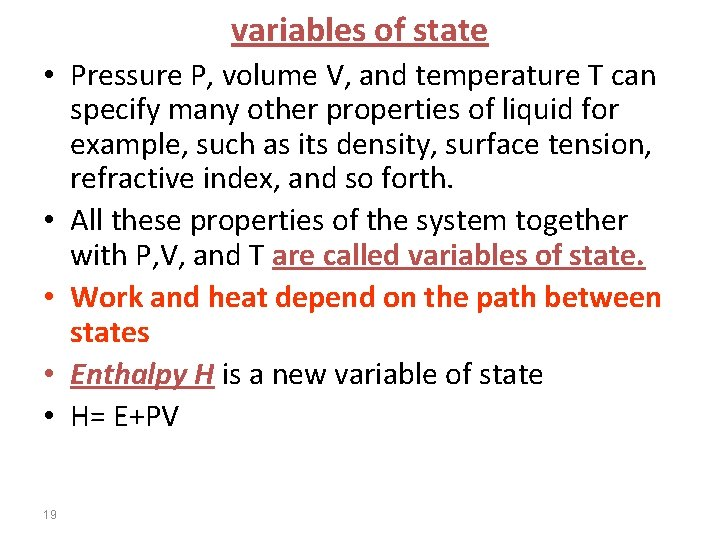variables of state • Pressure P, volume V, and temperature T can specify many other properties of liquid for example, such as its density, surface tension, refractive index, and so forth. • All these properties of the system together with P, V, and T are called variables of state. • Work and heat depend on the path between states • Enthalpy H is a new variable of state • H= E+PV 19• The energy or enthalpy change when the teaspoon of sugar is converted to CO 2 and H 2 O is the same whether sugar is burned in a reaction vessel or metabolized in human body, as long as the final states for the reaction are the same in the two cases. • It does not matter whether the conversion involves a direct reaction with O 2 (combustion) or multiple enzyme-catalyzed steps inside a human being • Table A. 6 table 2. 3 • The bond dissociation energy is the enthalpy at 25 o. C and 1 atm, for the reaction. • A-B (g) →A (g) + B(g) 20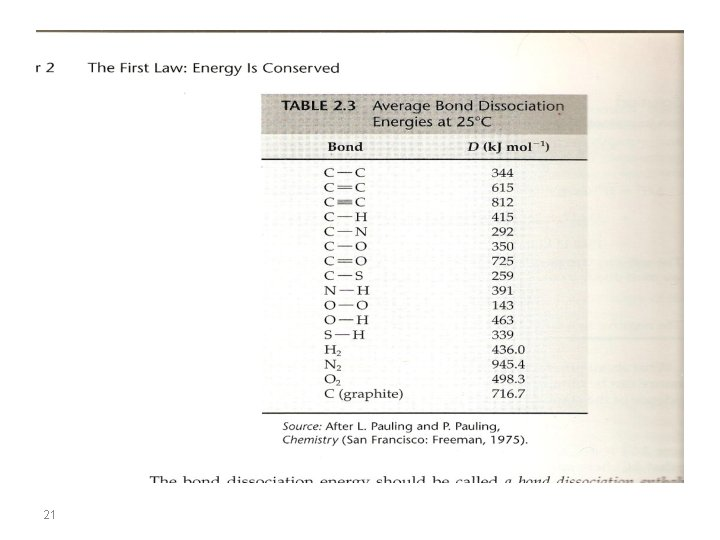2122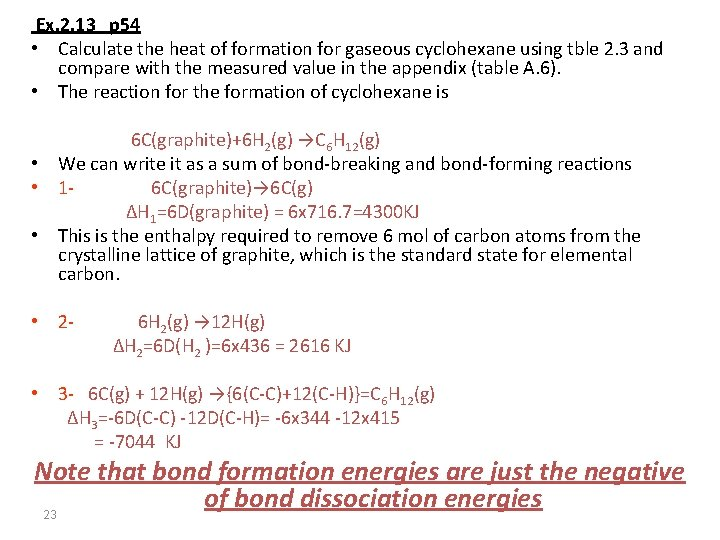Ex. 2. 13 p 54 • Calculate the heat of formation for gaseous cyclohexane using tble 2. 3 and compare with the measured value in the appendix (table A. 6). • The reaction for the formation of cyclohexane is 6 C(graphite)+6 H 2(g) →C 6 H 12(g) • We can write it as a sum of bond-breaking and bond-forming reactions • 16 C(graphite)→ 6 C(g) ΔH 1=6 D(graphite) = 6 x 716. 7=4300 KJ • This is the enthalpy required to remove 6 mol of carbon atoms from the crystalline lattice of graphite, which is the standard state for elemental carbon. • 2 - 6 H 2(g) → 12 H(g) ΔH 2=6 D(H 2 )=6 x 436 = 2616 KJ • 3 - 6 C(g) + 12 H(g) →{6(C-C)+12(C-H)}=C 6 H 12(g) ΔH 3=-6 D(C-C) -12 D(C-H)= -6 x 344 -12 x 415 = -7044 KJ Note that bond formation energies are just the negative of bond dissociation energies 23• ΔHf = ΔH 1 + ΔH 2 + ΔH 3 = (4300+ 2616)- 7044= -128 KJ The value in table A. 6 is -123. 15 KJ which is in good agreement. (molecule with normal bond) 24Ex. 2. 14 • Calculate the heat formation for gaseous benzene using table 2. 3 and compare with the measured value in table A. 6 in the appendix. • The reaction for benzene is 6 C(graphite)+3 H 2(g) →C 6 H 6(g) • 16 C(graphite)→ 6 C(g) ΔH 1=6 D(graphite) = 6 x 716. 7=4300 KJ • 2 - 3 H 2(g) → 6 H(g) ΔH 2=3 D(H 2 )=3 x 436 = 1308 KJ • 3 - 6 C(g) + 6 H(g) →{3(C-C)+3(C=C)+6(C-H)}=C 6 H 6(g) ΔH 3= - 3 D(C-C) -3 D(C=C) - 6(C-H) = -3 x 344 - 3 x 615 – 6 x 415 = -5367 KJ • ΔHf = ΔH 1 + ΔH 2 + ΔH 3 = (4300+ 1308) - 5367= 241 KJ The value in table A. 6 is 82. 93 i. e is about 158 KJ lower in enthalpy than would be expected. This energy is what we call the resonance energy. 25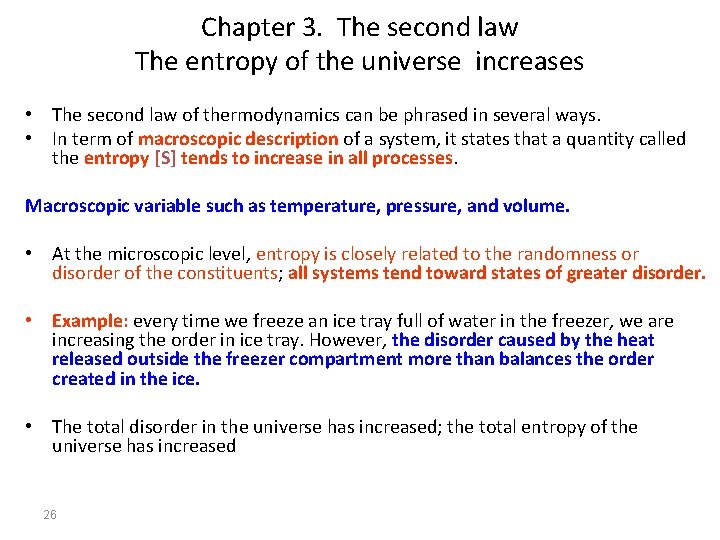Chapter 3. The second law The entropy of the universe increases • The second law of thermodynamics can be phrased in several ways. • In term of macroscopic description of a system, it states that a quantity called the entropy [S] tends to increase in all processes. Macroscopic variable such as temperature, pressure, and volume. • At the microscopic level, entropy is closely related to the randomness or disorder of the constituents; all systems tend toward states of greater disorder. • Example: every time we freeze an ice tray full of water in the freezer, we are increasing the order in ice tray. However, the disorder caused by the heat released outside the freezer compartment more than balances the order created in the ice. • The total disorder in the universe has increased; the total entropy of the universe has increased 26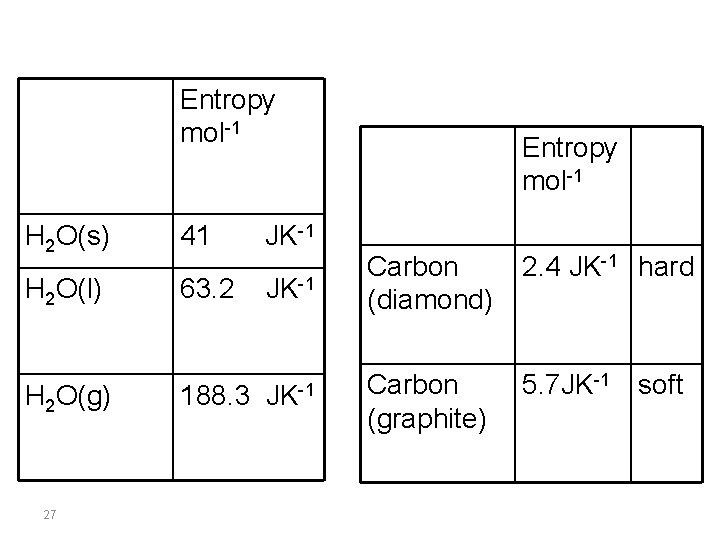Entropy mol-1 H 2 O(s) 41 JK-1 H 2 O(l) 63. 2 188. 3 H 2 O(g) 27 Entropy mol-1 JK-1 Carbon (diamond) 2. 4 JK-1 hard JK-1 Carbon (graphite) 5. 7 JK-1 soft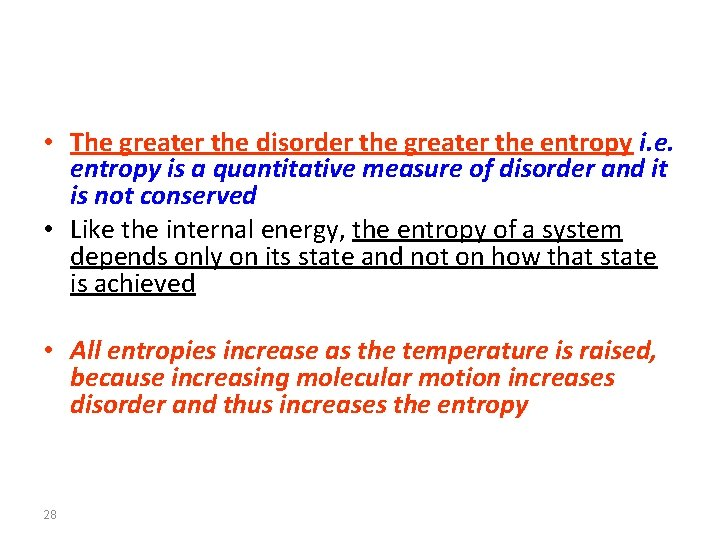• The greater the disorder the greater the entropy i. e. entropy is a quantitative measure of disorder and it is not conserved • Like the internal energy, the entropy of a system depends only on its state and not on how that state is achieved • All entropies increase as the temperature is raised, because increasing molecular motion increases disorder and thus increases the entropy 28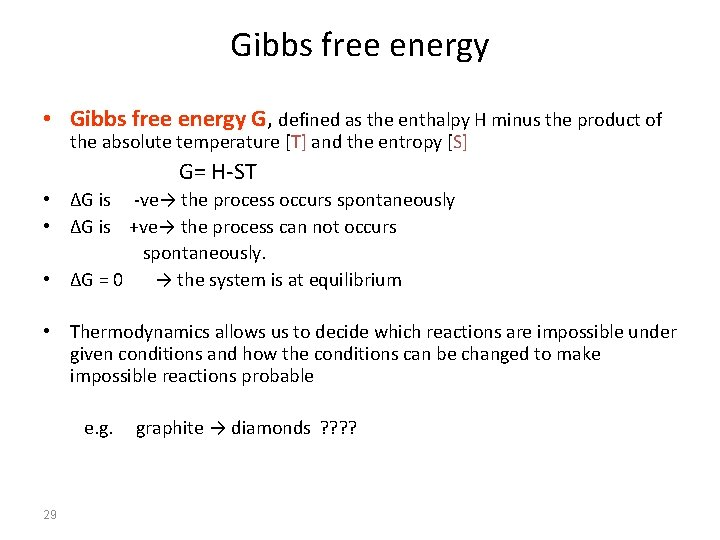Gibbs free energy • Gibbs free energy G, defined as the enthalpy H minus the product of the absolute temperature [T] and the entropy [S] G= H-ST • ΔG is -ve→ the process occurs spontaneously • ΔG is +ve→ the process can not occurs spontaneously. • ΔG = 0 → the system is at equilibrium • Thermodynamics allows us to decide which reactions are impossible under given conditions and how the conditions can be changed to make impossible reactions probable e. g. 29 graphite → diamonds ? ?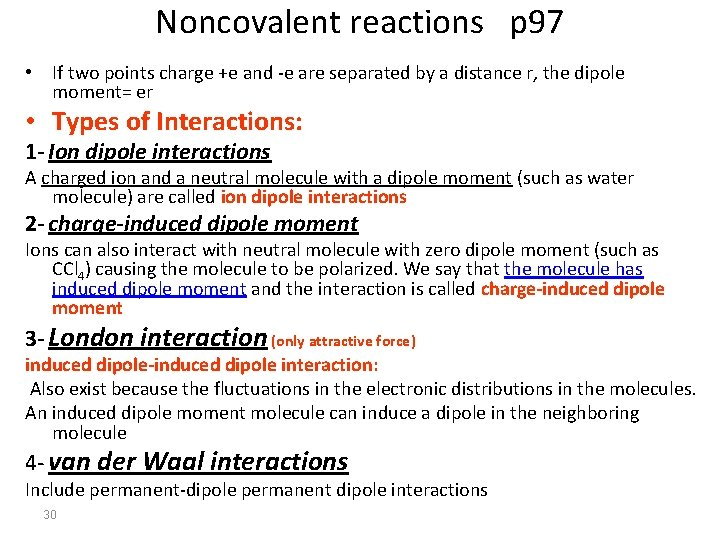Noncovalent reactions p 97 • If two points charge +e and -e are separated by a distance r, the dipole moment= er • Types of Interactions: 1 - Ion dipole interactions A charged ion and a neutral molecule with a dipole moment (such as water molecule) are called ion dipole interactions 2 - charge-induced dipole moment Ions can also interact with neutral molecule with zero dipole moment (such as CCl 4) causing the molecule to be polarized. We say that the molecule has induced dipole moment and the interaction is called charge-induced dipole moment 3 - London interaction (only attractive force) induced dipole-induced dipole interaction: Also exist because the fluctuations in the electronic distributions in the molecules. An induced dipole moment molecule can induce a dipole in the neighboring molecule 4 - van der Waal interactions Include permanent-dipole permanent dipole interactions 30Noncovalent reactions p 97 The London-van der Waal forces can become specific when carful fitting of molecules is involved. Binding of a particular substrate to an enzyme, antigen-antibody binding, and the function of specific membrane lipids may be dominated by The London-van der Waal interactions 5 - The hydrogen bond Is one of the important bonds that determines the three dimentional structure of proteins and nucleic acids. O-H. . O, N-H. . N, N-H. . O 6 - Hydrophobic interactions (fear of water) It is important in aqueous solutions. Water molecules have a strong attraction for each other (as a consequence of hydrogen-bond formation) The oxygen atom of most molecules of liquid water is hydrogen-bonded to two hydrogen atoms of two other water molecules and also the hydrogen atoms Therefore, the molecules of liquid water form a mobile network. The network is not a rigid one, and change in neighbors occurs rapidly because of thermal motions 31Noncovalent reactions • Water-soluble proteins, polar lipid molecules form bilayer sheets or membranes in water, in which the hydrocarbon proteins are buried inside and the polar or charged proteins are on the surface exposed to water. Such molecules are called amphiphilic • Hydrophobic interactions are characterized by low enthalpy changes and are entropy driven • We should note that hydrophobic interaction is a term that we use to describe the combined effects of London, van der Waals, and hydrogen-bonding interactions in certain processes in aqueous solutions 32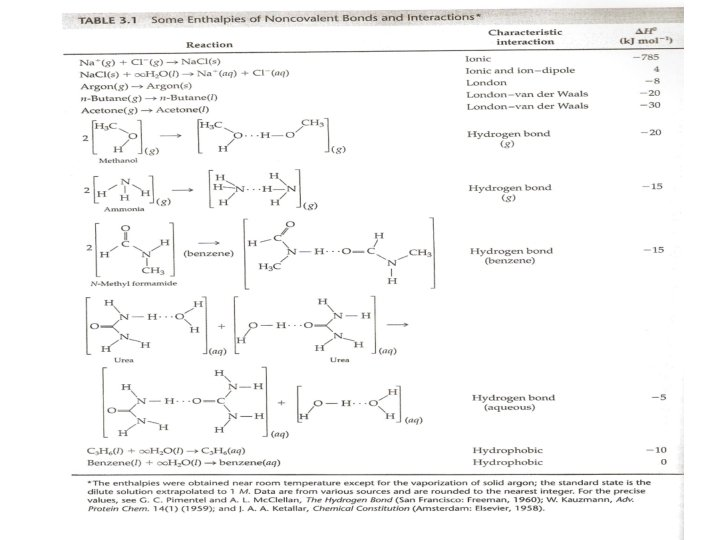33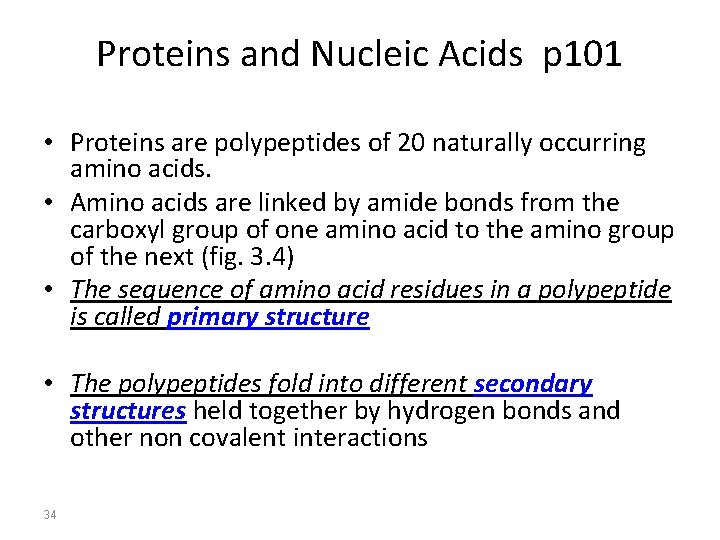Proteins and Nucleic Acids p 101 • Proteins are polypeptides of 20 naturally occurring amino acids. • Amino acids are linked by amide bonds from the carboxyl group of one amino acid to the amino group of the next (fig. 3. 4) • The sequence of amino acid residues in a polypeptide is called primary structure • The polypeptides fold into different secondary structures held together by hydrogen bonds and other non covalent interactions 34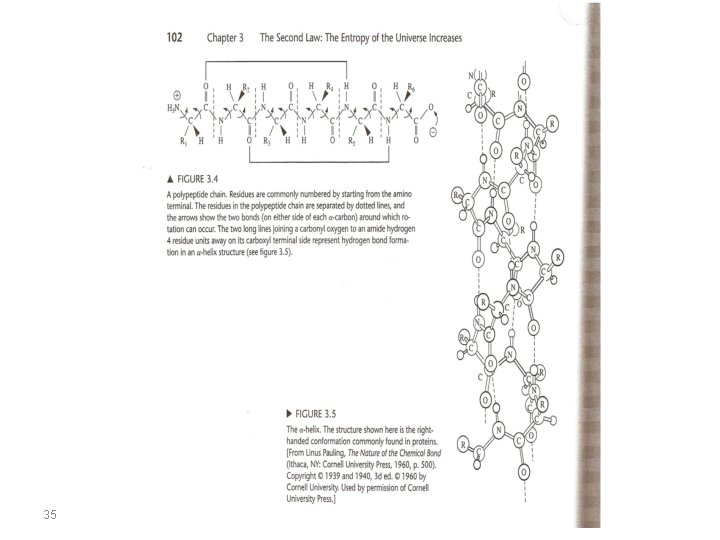35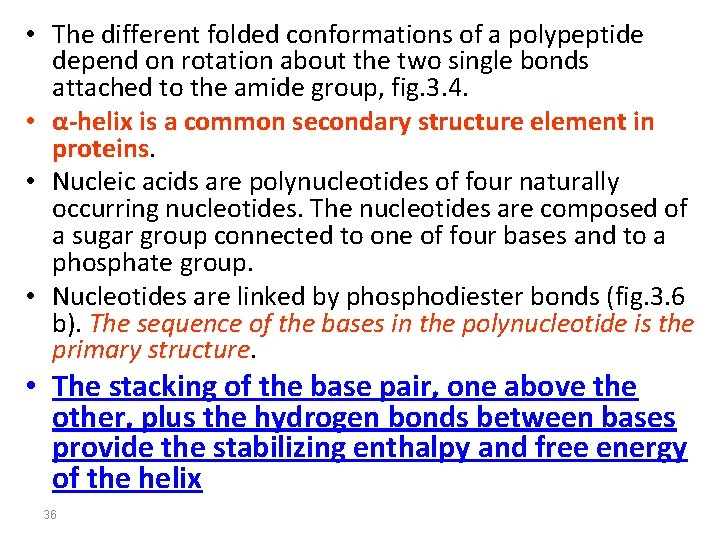• The different folded conformations of a polypeptide depend on rotation about the two single bonds attached to the amide group, fig. 3. 4. • α-helix is a common secondary structure element in proteins. • Nucleic acids are polynucleotides of four naturally occurring nucleotides. The nucleotides are composed of a sugar group connected to one of four bases and to a phosphate group. • Nucleotides are linked by phosphodiester bonds (fig. 3. 6 b). The sequence of the bases in the polynucleotide is the primary structure. • The stacking of the base pair, one above the other, plus the hydrogen bonds between bases provide the stabilizing enthalpy and free energy of the helix 3637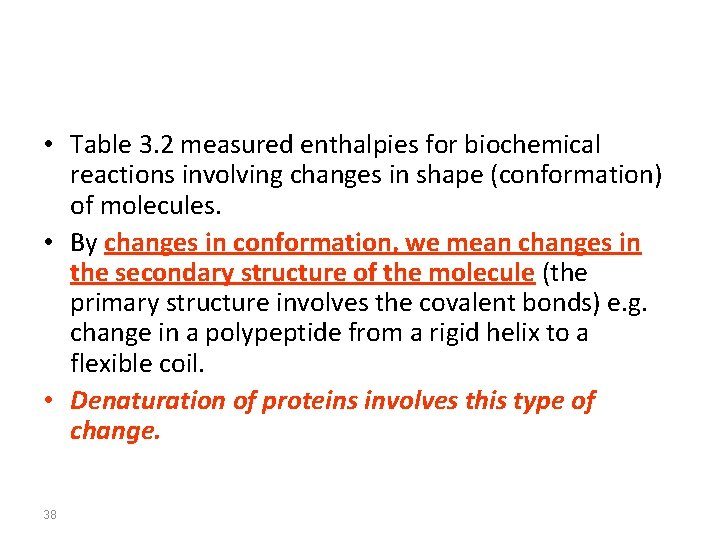• Table 3. 2 measured enthalpies for biochemical reactions involving changes in shape (conformation) of molecules. • By changes in conformation, we mean changes in the secondary structure of the molecule (the primary structure involves the covalent bonds) e. g. change in a polypeptide from a rigid helix to a flexible coil. • Denaturation of proteins involves this type of change. 3839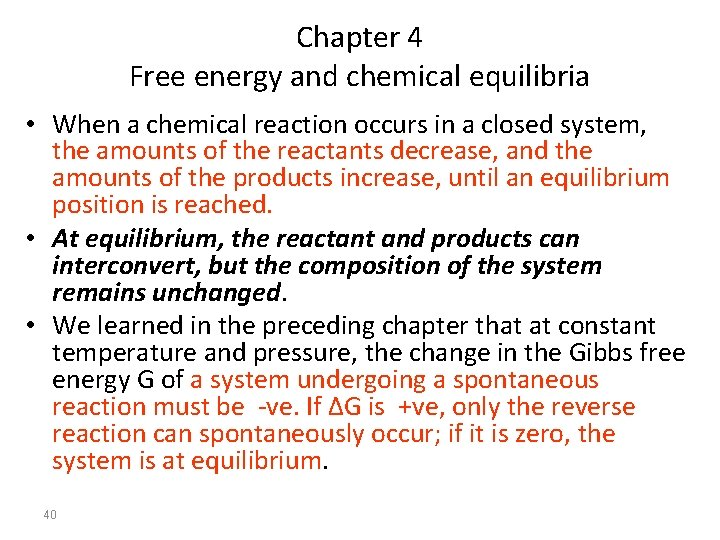Chapter 4 Free energy and chemical equilibria • When a chemical reaction occurs in a closed system, the amounts of the reactants decrease, and the amounts of the products increase, until an equilibrium position is reached. • At equilibrium, the reactant and products can interconvert, but the composition of the system remains unchanged. • We learned in the preceding chapter that at constant temperature and pressure, the change in the Gibbs free energy G of a system undergoing a spontaneous reaction must be -ve. If ΔG is +ve, only the reverse reaction can spontaneously occur; if it is zero, the system is at equilibrium. 40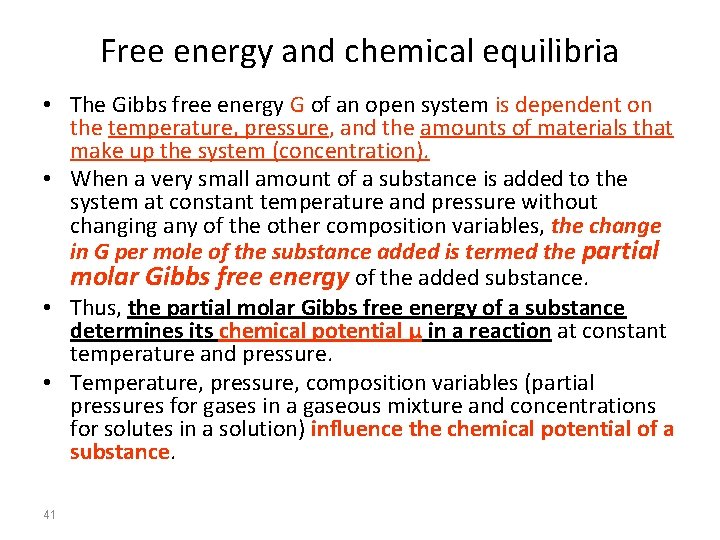Free energy and chemical equilibria • The Gibbs free energy G of an open system is dependent on the temperature, pressure, and the amounts of materials that make up the system (concentration). • When a very small amount of a substance is added to the system at constant temperature and pressure without changing any of the other composition variables, the change in G per mole of the substance added is termed the partial molar Gibbs free energy of the added substance. • Thus, the partial molar Gibbs free energy of a substance determines its chemical potential μ in a reaction at constant temperature and pressure. • Temperature, pressure, composition variables (partial pressures for gases in a gaseous mixture and concentrations for solutes in a solution) influence the chemical potential of a substance. 41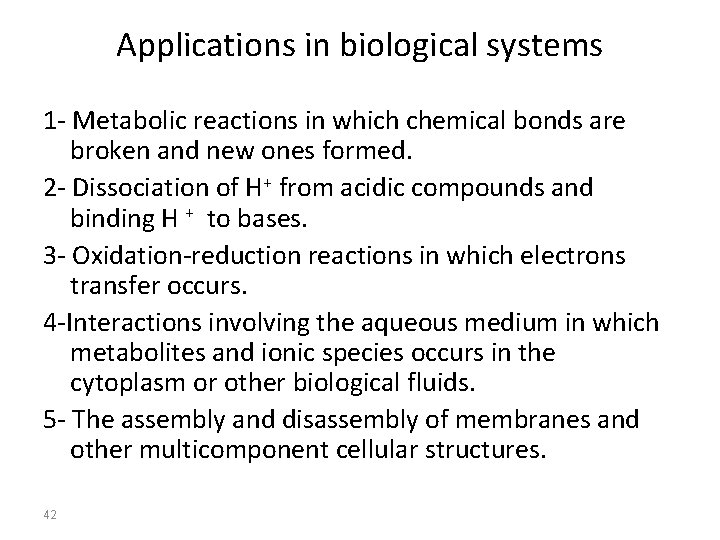Applications in biological systems 1 - Metabolic reactions in which chemical bonds are broken and new ones formed. 2 - Dissociation of H+ from acidic compounds and binding H + to bases. 3 - Oxidation-reduction reactions in which electrons transfer occurs. 4 -Interactions involving the aqueous medium in which metabolites and ionic species occurs in the cytoplasm or other biological fluids. 5 - The assembly and disassembly of membranes and other multicomponent cellular structures. 42Chapter 5 Free energy and physical equilibria • Applications • Membrane and transport • Most biological membranes consist of a lipid bilayer that contains proteins and other molecules that serve as recognition sites, signal transmitters, or ports of entrance and exit. • Membranes are so thin that they are often considered to be two-dimensional phases. • The thermodynamic properties of the membrane are then described in terms of surface properties, such as the surface chemical potential and the surface tension or pressure. 43• Membranes permit the controlled transport of molecules and signals between the inside and outside. • Difference between the inside and outside of a cell influence the exchange of metabolites and electrical signals, the flow of heat, and changes in shape. 1 - Temperature differences causes heat flow. 2 - Pressure differences cause changes in shape. 3 - Electrochemical potential differences cause molecular transport and electrical signals. 44Ligand binding p 188 • Noncovalent interactions that bind ligands like O 2 to hemoglobin, substrate to enzymes, and complementary strands of DNA or RNA to one another underlie essential dynamic processes in living cells. • Equilibrium dialysis provides a method of exploring the binding between macromolecules and small ligand molecules. • In equilibrium dialysis a semipermeable membrane allows a ligand to reach equilibrium between two phases, one of which contains a macromolecule. The difference in concentrations of ligand on opposite sides of the membrane depends on the interaction of ligands and macromolecule. 45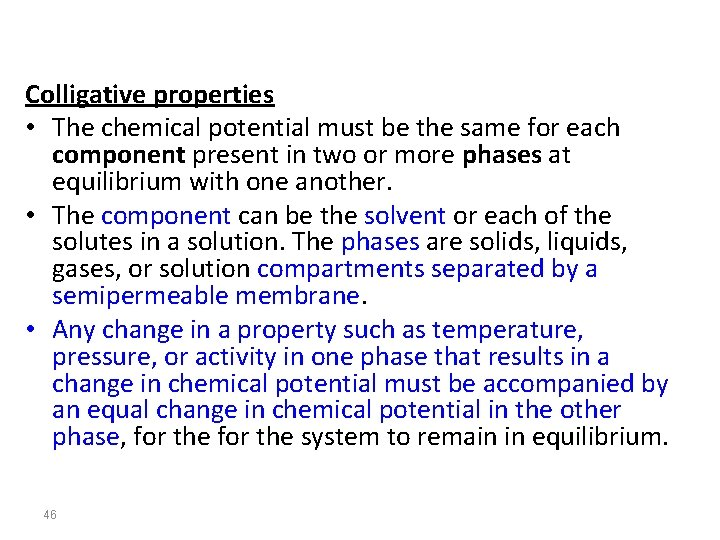Colligative properties • The chemical potential must be the same for each component present in two or more phases at equilibrium with one another. • The component can be the solvent or each of the solutes in a solution. The phases are solids, liquids, gases, or solution compartments separated by a semipermeable membrane. • Any change in a property such as temperature, pressure, or activity in one phase that results in a change in chemical potential must be accompanied by an equal change in chemical potential in the other phase, for the system to remain in equilibrium. 46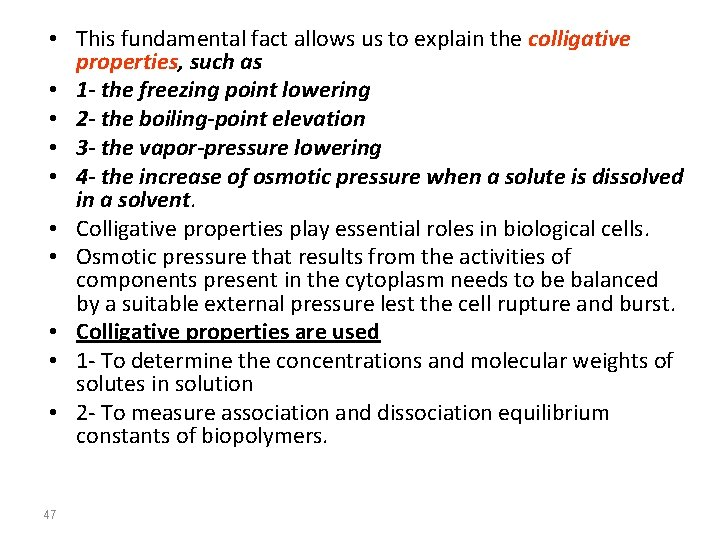• This fundamental fact allows us to explain the colligative properties, such as • 1 - the freezing point lowering • 2 - the boiling-point elevation • 3 - the vapor-pressure lowering • 4 - the increase of osmotic pressure when a solute is dissolved in a solvent. • Colligative properties play essential roles in biological cells. • Osmotic pressure that results from the activities of components present in the cytoplasm needs to be balanced by a suitable external pressure lest the cell rupture and burst. • Colligative properties are used • 1 - To determine the concentrations and molecular weights of solutes in solution • 2 - To measure association and dissociation equilibrium constants of biopolymers. 47• • • 48 Phase equilibria The transfer of a chemical from one phase to another is illustrated by : 1 -the evaporation of liquid water into vapor phase 2 - the heat removed from our bodies by evaporation of sweat is essential to survival in hot climates. 3 - the transport of ions from inside a cell to outside, which is vital to nerve conduction. (we can consider the inside and the outside of the cell as two different phases).